# Research on Heat-Transfer and Three-Dimensional Characteristics of Natural Convection in A Small Cavity with Heat Sources

Research on Heat-Transfer and Three-Dimensional Characteristics of Natural Convection in A Small Cavity with Heat Sources

Naiyan ZhanYue XuZhiyun Wang

School of Municipal and Environment Engineering Department, Jilin Jianzhu University, Changchun, Jilin 130118, P.R.China

College of Power Engineering, University of Shanghai for Science and Technology, Shanghai 200031, P.R. China

Corresponding Author Email:
zhannaiyan@jlju.edu.cn
Page:
59-66
|
DOI:
http://doi.org/10.18280/ijht.330308
N/A
|
Accepted:
N/A
|
Published:
30 September 2015
| Citation

OPEN ACCESS

Abstract:

To address the cooling problem for compact electronic equipment, numerical simulations were conducted using the SIMPLE algorithm with a QUICK scheme. Natural convection was numerically simulated in a small cavity with one, two, or three heat sources. The temperature field, flow field, and three-dimensional characteristics of the system were studied. The results showed that the number of vortices and the air temperature increased with more heat sources. With lower Rayleigh number, heat transfer was better in a square cavity than in a rectangular one. The average Nusselt number at the top plate was larger with the heat source close to the center rather than close to the boundary. The flow and heat-transfer constraints were satisfied by the simulation. Heat transfer was better with the heat source close to the center rather than close to the boundary. The X=Lx/2 temperature fields were similar to two-dimensional temperature fields, and therefore the three-dimensional model could be replaced by a two-dimensional model.

Keywords:

Small cavity, Heat sources, Mechanism, Three-dimensional characteristics.

1. Introduction

Compact electronic equipment is evolving rapidly as high-tech products pervade our world. They are present in every aspect of human life and include devices such as plasma TVs, panel computers, mobile phones, digital set-top boxes, and portable CD players. Heat dissipation is becoming more and more difficult because electronic equipment is continually evolving towards high-density packing and multiple functions . With more and more mirco-electronic devices, efficient and for heat generated to ease and cooling gradually become hot and difficult research  .If heat dissipation is insufficient, electronic components cannot function properly and will fail. Excess heat shortens the service life of equipment and can even damage it. According to statistics, 55% of electronic equipment failures are caused by high temperature . Therefore, effective heat dissipation in electronic equipment is crucial to improving product reliability.

To dissipate heat from compact electronic equipment, aluminum sheets and fans cannot be used because of limited space. Forced flow cannot be permitted because of the effect on signal transmission (especially in outdoor communication equipment) to avoid noise mixing. Hence, heat dissipation by natural convection becomes the inevitable choice. Natural convection is a classic and convenient heat-dissipation method. Its advantages are simple structure, low cost, safety and reliability, and absence of noise and vibration .

Closed plurality of isolated square cavity natural convection heat transfer in many heat transfer approximation analog electronic equipment, therefore, to understand the closed square cavity multiple isolated natural convection heat transfer plates law, for the thermal design of electronic equipment is of great significance .

A number of studies have been published on this topic. Man studied heat transfer by natural convection in a square cavity with a heat source .Yang et al. Analyzed analyzed a method for processing two different physical parameters of the closed cavity natural convection under different temperature difference numerical calculation . Song Shanshan and Guo Xueyan used three different densities approach closing party on natural convection heat transfer numerical simulation . Pei et al applied into a river SIMPLE algorithm Numerical simulation of the solid skeleton porous medium heat steady non-Darcy square cavity convection, the effects of different conditions other cavity natural convection and heat transfer . Manab studied the influence of the tilt angle . Sharif simulated heat transfer by natural convection in a square cavity with a heat source and confirmed the influence of the lateral walls on natural convection . Dong et al. confirmed the influence of cavity geometry . Wang conducted experiments to study natural convection in an inclined square cavity with isolated plates. These experiments confirmed the influence of the plate position, the cavity inclination angle, and the Rayleigh number on heat transfer . Jiang conducted experiments on natural convection in a rectangular cavity with scattered heat sources. These showed that the plate temperature and heat-transfer coefficient of scattered heat sources were related only to the self-heating power and the heat-transfer conditions .

All these researchers mainly studied natural convection in a cavity with one heat source. Few studies have been performed on natural convection in a cavity with more than heat source. In this paper, natural convection was numerically simulated in a small cavity with one or more heat sources using the SIMPLE algorithm with a QUICK scheme. Heat-transfer mechanisms and their three-dimensional characteristics were investigated.

2. Mathematical Formulation and Solution Method

2.1 Problem and mathematical formulation

The problem, as shown in Fig. 1, involves three-dimensional natural convection and heat transfer in a small cavity with one or more heat sources. The temperature of the heat sources inside the cavity is denoted by Th. The bottom and top plates are cooled isothermally and have temperature Tc (Th > Tc). The air is considered as a Boussinesq fluid with a Prandtl number of 0.701. The lateral walls are adiabatic. The aspect ratio along the z-axis is invariable, and the aspect ratios along the x-axis (Lx=L:H) are Lx=0.25, Lx=0.5, Lx=1, Lx=2, Lx=4, and Lx=8 respectively.

The size and temperature of the heat sources are assumed to be approximately the same, but the number of heat sources is variable.

## 1.png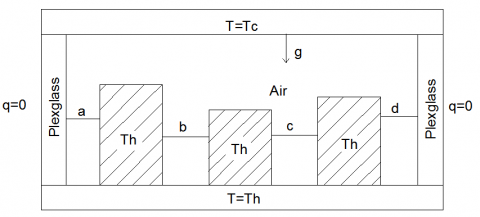Figure 1. Cavity geometry

The dimensionless governing equations for the conservation of mass, momentum, and energy can be expressed as follows:

$\frac{\partial U}{\partial \tau}+U \frac{\partial U}{\partial X}+V \frac{\partial U}{\partial Y}+W \frac{\partial U}{\partial Z}=-\frac{\partial P}{\partial X}+\sqrt{\frac{P r}{R a}}\left(\frac{\partial^{2} U}{\partial X^{2}}+\frac{\partial^{2} U}{\partial Y^{2}}+\frac{\partial^{2} U}{\partial Z^{2}}\right)$    (1)

$\frac{\partial V}{\partial \tau}+U \frac{\partial V}{\partial X}+V \frac{\partial V}{\partial Y}+W \frac{\partial V}{\partial Z}=-\frac{\partial P}{\partial Y}+\sqrt{\frac{P r}{R a}}\left(\frac{\partial^{2} V}{\partial X^{2}}+\frac{\partial^{2} V}{\partial Y^{2}}+\frac{\partial^{2} V}{\partial Z^{2}}\right)+\Theta$   (2)

$\frac{\partial W}{\partial \tau}+U \frac{\partial W}{\partial X}+V \frac{\partial W}{\partial Y}+W \frac{\partial W}{\partial Z}=-\frac{\partial P}{\partial Z}+\sqrt{\frac{P r}{R a}}\left(\frac{\partial^{2} W}{\partial X^{2}}+\frac{\partial^{2} W}{\partial Y^{2}}+\frac{\partial^{2} W}{\partial Z^{2}}\right)$  (3)

$\frac{\partial \Theta}{\partial \tau}+U \frac{\partial \Theta}{\partial X}+V \frac{\partial \Theta}{\partial Y}+W \frac{\partial \Theta}{\partial Z}=\frac{1}{\sqrt{R a P r}}\left(\frac{\partial^{2} \Theta}{\partial X^{2}}+\frac{\partial^{2} \Theta}{\partial Y^{2}}+\frac{\partial^{2} \Theta}{\partial Z^{2}}\right)$  (4)

$\frac{\partial U}{\partial X}+\frac{\partial V}{\partial Y}+\frac{\partial W}{\partial Z}=0$     (5)

2.2 Boundary conditions

The boundary conditions in dimensionless form are the following:

$X=0, U=V=W=0, \frac{\partial \Theta}{\partial X}=0$   (6)

$X=L_{x}, U=V=W=0, \frac{\partial \Theta}{\partial X}=0$     (7)

$Y=0, U=W=0, \Theta=0$   (8)

$Y=1, U=W=0, \Theta=0$    (9)

$Z=0, U=V=W=0, \frac{\partial \Theta}{\partial Z}=0$    (10)

$Z=L_{z}, U=V=W=0, \frac{\partial \Theta}{\partial Z}=0$     (11)

Where the reference velocity is UR=(Ra·Pr)1/2·α/H, the time is τ=t·UR/H, and the pressure is P=p/(ρ·UR2). The temperature is θ=(T-Tc)/△T, where △T=Th-Tc. α is the thermal diffusivity, and ρ is the fluid density. The Rayleigh number, which determines the strength of the convection vortices, is defined as Ra=T·H3Pr/γ2. The coefficient of thermal expansion is defined as:

$\beta=-\left[\frac{1}{\rho}(\partial \rho / \partial T)\right]_{T_{0}}$

2.3 Solution method

The governing equations were solved in primitive variables, using a three-dimensional staggered grid based on the control volume method. The SIMPLE algorithm with a QUICK scheme was used to solve the coupled heat-transfer and flow problem.

To verify the SIMPLE algorithm with the QUICK scheme, a few examples were simulated and compared with previously obtained experimental and simulated results. Table 1 shows a comparison is shown between Nusselt numbers obtained at different Rayleigh numbers (the grid is 100×100×100) and benchmark solutions given in the literature . As shown in Table 1, the results obtained in this research are consistent with the NCMARKATOS benchmark solutions, with an error of less than 2%.

The number of grid cells and the time step were determined for the purposes of this research. Each grid was divided into equal partitions, and the number of grid cells was 100(x) × 100(y) × 100(z). Dimensionless time was 0.01. In this research, a 200(x) × 200(y) × 200(z) grid and a dimensionless time of 0.001 were used for verification. The relative differences between the Nusselt numbers obtained in this research and those obtained from the grids were less than 0.5%.

Table 1. Comparison between the solutions determined in this research and the NCMARKATOS benchmark solutions 

 Ra 103 104 105 Nuc (reference) 1.108 2.201 4.430 Nuc (this article) 1.117 2.238 4.471 Error 0.81% 1.68% 0.93%
3. Results and Discussion

3.1 Description of simulated results

In this experiment, the aspect ratio along the x-axis was 4(Lx=4). The ratio of the heat-source height to the cavity height was 0.4. The initial condition was zero. The three cases were investigated with one, two, and three heat sources. The temperature and velocity vector fields observed were described at Ra=3000 and are shown in Figs. 2, 3, and 4.

## 2a.png(a) Temperature field

## 2b.png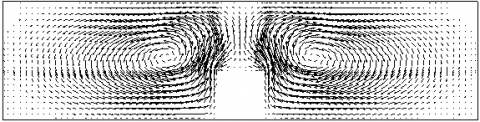(b) Velocity vector field

Figure 2. One heat source.

## 3a.png(a) Temperature field

## 3b.png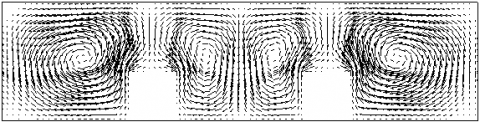(b) Velocity vector field

Figure 3. Two heat sources

## 4a.png(a) Temperature field

## 4b.png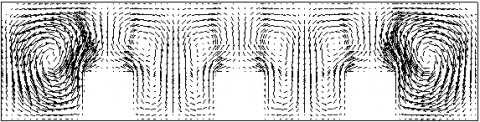(b) Velocity vector field

Figure 4. Three heat sources

Two symmetrical vortices appeared when one heat source was present in the cavity (Fig. 2). When two heat sources were present in the cavity, four vortices appeared (Fig. 3). These vortices were thin close to the center, but fat near the boundaries. With three heat sources, six vortices appeared (also shown in Fig. 3). These vortices were also thin close to the center, but fat near the boundaries. The number of vortices and the temperature clearly increased with a larger number of heat sources.

3.2 Influence of cavity size on flow field and heat transfer

Six cases were investigated, with aspect ratios Lx=0.25, 0.5, 1, 2, 4, and 8. The ratio of the heat-source height to the cavity height was 0.4. The initial condition was zero, and the number of heat sources was one. The velocity vector fields are illustrated in Fig. 5 for Ra=3000. The average Nusselt numbers at the top plate are shown in Fig. 6.

## 5a.png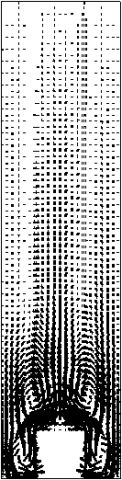(a) Lx=0.25

## 5b.png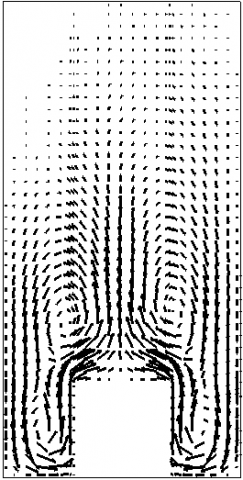(b) Lx=0.5

## 5c.png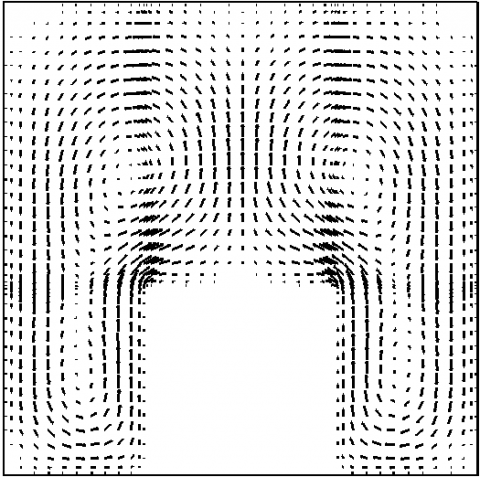(c) Lx=1

## 5d.png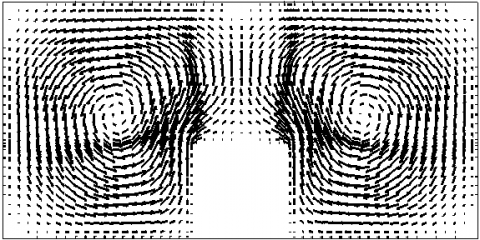(d) Lx=2

## 5e.png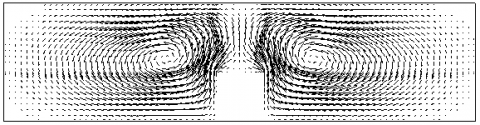(e) Lx=4

## 5f.png(f) Lx=8

Figure 5. Velocity vector field for varying geometric size

## 6.png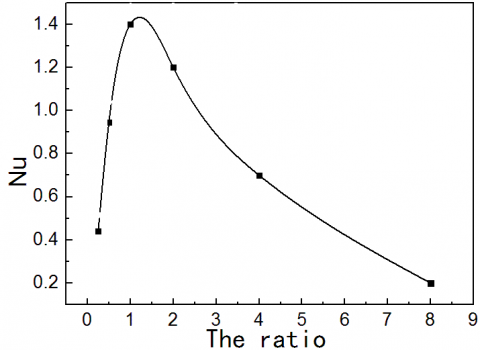Figure 6. Average Nusselt number for varying ratio.

As shown in Fig. 6, the average Nusselt numbers at the top plate first increased and then decreased as the aspect ratios increased. The average Nusselt number at the top plate was greatest at Lx=1. The flow and the heat transfer first became stronger and then weaker. The flow and the heat transfer were also strongest at Lx=1.

Two symmetrical vortices quickly became concentrated on the heat source with the flow at Lx=1 (as shown in Fig. 5(c)). The heat-transfer effect was strong. The two vortices became prolate and flowed weakly at Lx=4 and Lx=8 (Figs. 5(e) and 5(f)). The heat-transfer effect in this case was relatively weak. At Lx=0.5 and Lx=0.25, two symmetrical vortices were concentrated on the heat source (Figs. 5(a) and 5(b)). They were slender and flowed weakly when they arrived at the top plate. The heat-transfer effect in this case was relatively weak. At lower Rayleigh number, the heat-transfer effect was better in a square cavity than in a rectangular one.

3.3 Influence of heat sources size on flow field and heat transfer

In this experiment, the aspect ratio along the x-axis was 2(Lx=2). The number of heat sources was one. Three cases were investigated, with the ratio of the heat-source height to the cavity height varying from 0.1 to 0.9 The average Nusselt numbers at the top plate are shown in Fig. 7.

## 7.pngFigure 7. Average Nusselt number for varying ratio

As shown in Fig. 7, the average Nusselt numbers at the top plate first increased as the ratio of the heat-source height to the cavity height increased. The flow and the heat transfer became stronger.

3.4 Influence of heat source location on fluid flow and heat transfer

In this experiment, the aspect ratio along the x-axis was 2(Lx=2). The number of heat sources was one. The ratio of the heat-source height to the cavity height was 0.4. The initial condition was zero. The eight cases were investigated, with the location of the heat source varying from 0.1 to 0.8. The temperature fields and the average Nusselt number at the top plate were observed. The temperature fields are shown in Fig. 8, and the average Nusselt numbers at the top plate are shown in Fig. 9.

## 8a.png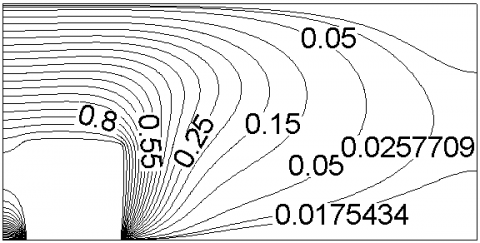(a) 0.1

## 8b.png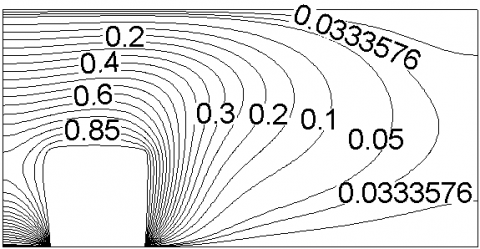(b) 0.2

## 8c.png(c) 0.3

## 8d.png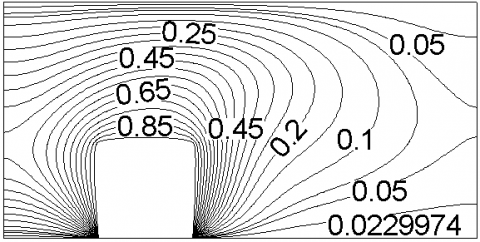(d) 0.4

## 8e.png(e) 0.5

## 8f.png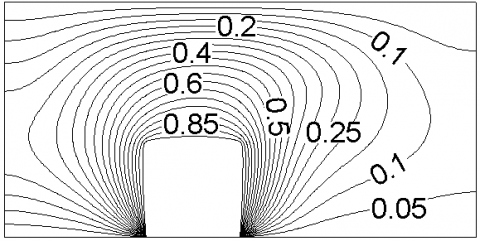(f) 0.6

## 8g.png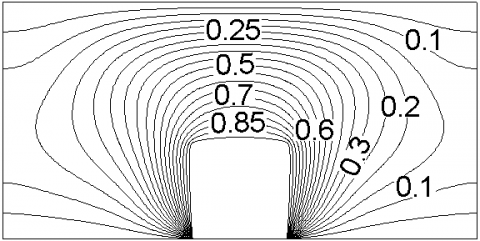(g) 0.7

## 8h.png(h) 0.8

Figure 8. Temperature field for varying location of the heat source

As shown in Fig. 8, the temperature was higher near the boundary and lower close to the center when the heat source was close to the center.

## 9.png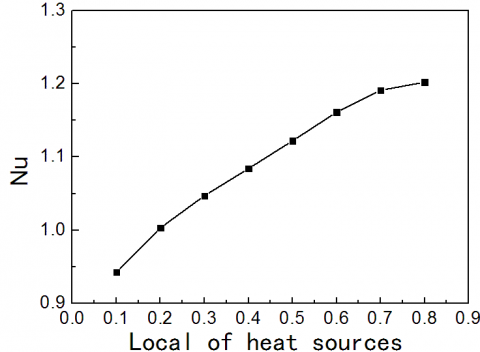Figure 9. Average Nusselt number for varying location of the heat source.

As shown in Fig. 9, the average Nusselt number at the top plate was higher when the heat source was close to the center than when it was near the boundary. The flow and the heat transfer were strong. The heat-transfer effect was better when the heat source was close to the center, rather than near the boundary.

3.5 Influence of the number of heat sources on flow and heat transfer

In this experiment, flow and heat transfer were studied in a cavity with an aspect ratio along the x-axis of 4. The ratio of the heat-source height to the cavity height was 0.4. The initial condition was zero. The three cases were investigated with one, two, and three heat sources. The average Nusselt number at the top plate is shown in Fig. 10.

## 10.png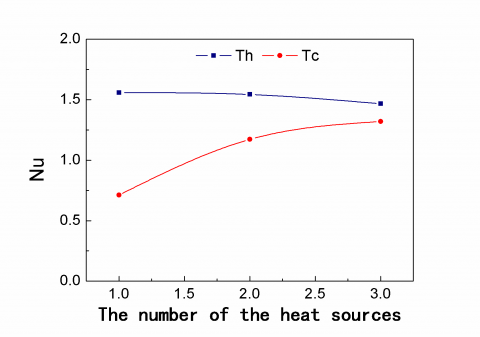Figure 10. Average Nusselt number with varying number of heat sources

As shown in Fig. 10, the average Nusselt numbers at the top plate decreased with increasing number of heat sources when the temperature of the bottom plate was Th. Flow and heat transfer were relatively weak. The average Nusselt numbers at the top plate increased with more heat sources when the temperature of the bottom plate was Tc. Flow and heat-transfer constraints were satisfied.

The heat sources did not benefit from rising flow when the temperature of the bottom plate was Th. The effect was greater with a larger number of heat sources. The heat sources encouraged flow to rise when the temperature of the bottom plate was Tc, thus strengthening natural convection.

3.6 Influence of boundary conditions on flow field and heat transfer

The six cases were investigated with aspect ratios of Lx=0.25, 0.5, 1, 2, 4, and 8. The ratio of the heat-source height to the cavity height was 0.4. The initial condition was zero, and the number of heat sources was one. The temperatures of the bottom plate were Th and Tc respectively under the two boundary condition cases. The average Nusselt numbers at the top plate are presented in Fig. 11.

## 11.pngFigure 11. Average Nusselt number for different ratios.

The variation trends of the average Nusselt number were similar at Lx 1 when the temperatures of the bottom plate were Th and Tc respectively under the two boundary condition cases. The average Nusselt number at the top plate increased as the ratio increased. Flow and heat-transfer constraints were satisfied. The trends in the average Nusselt number varied differently when Lx > 1. The average Nusselt number at the top plate decreased with increasing ratio when the temperature of the bottom plate was Tc, but increased with increasing ratio when the temperature of the bottom plate was Th. The heat-transfer effect was better in a square cavity than in a rectangular cavity under all boundary conditions.

3.7 Three-dimensional characteristics

This section describes the investigations using the two- and three-dimensional models. Flow patterns and three-dimensional characteristics were studied. Assuming a minimal effect of the lateral boundaries on the central section, the central portions parallel to the two axes were observed in the three-dimensional simulated results. The results are shown in Figs. 12-14. The aspect ratios along the z-axis were invariable (Lz=2), and the aspect ratios along the x-axis were Lx=1, Lx=2, Lx=4, and Lx=8 respectively. The Rayleigh number was 3×103.

## 12a.png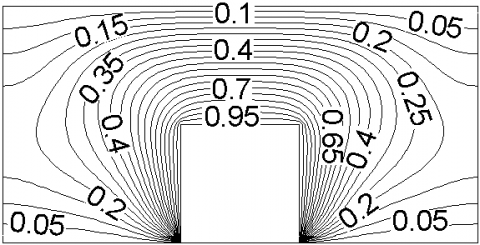(a) Two-dimensional

## 12b.png(b) Temperature of X=Lx/2

## 12c.png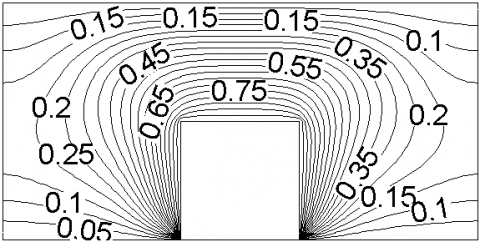(c) Temperature of Z=Lz/2

Figure 12. Temperature field at Lx=2

## 13a.png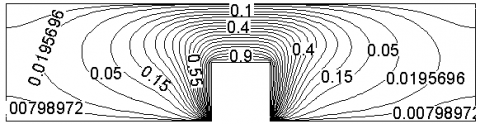(a) Two-dimensional

## 13b.png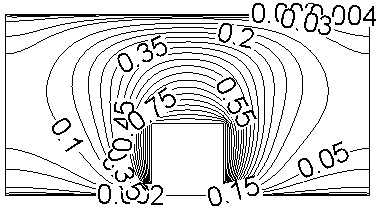(b) Temperature of X=Lx/2

## 13c.png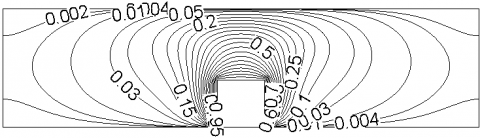(c) Temperature of Z=Lz/2

Figure 13. Temperature field at Lx=4

## 14a.png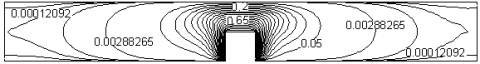(a) Two-dimensional

## 14b.png(b) Temperature of X=Lx/2

## 14c.png(c) Temperature of Z=Lz/2

Figure 14. Temperature field at Lx=8

As shown in Fig. 12-14, the X=Lx/2 temperature fields are the same as the two-dimensional temperature fields.

The two-dimensional average Nusselt number, the three-dimensional average Nusselt number of all sections parallel to the z-axis, and the three-dimensional average Nusselt number were compared for aspect ratios of 1, 2, 4, and 8. As shown in Fig. 15, the three-dimensional average Nusselt number of all sections parallel to the z-axis $\overline{N u_{i}}$ is denoted by ‘a’. The two-dimensional average Nusselt number $\overline{N u}_{2 d}$ is denoted by ‘b’. The three-dimensional average Nusselt number $\overline{N u}_{3 d}$ is denoted by ‘c’. Some conclusions can be drawn from this. $\overline{N u_{i}}$ is similar to $\overline{N u}_{2 d}$, and the difference between $\overline{N u}_{2 d}$ and $\overline{N u}_{3 d}$ is less than 8%. Therefore, the three-dimensional model can be replaced by the two-dimensional model.

## 15a.png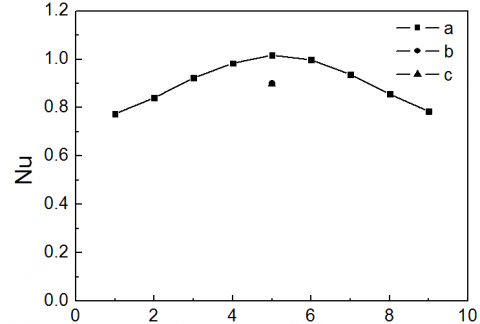(a) Lx=1

## 15b.png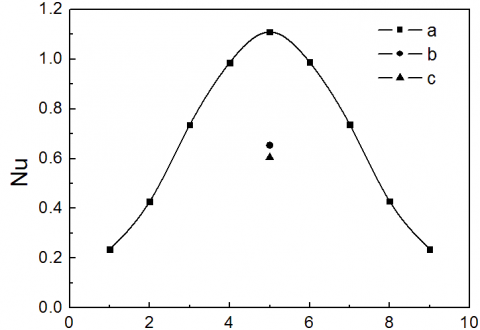(b) Lx=2

## 15c.png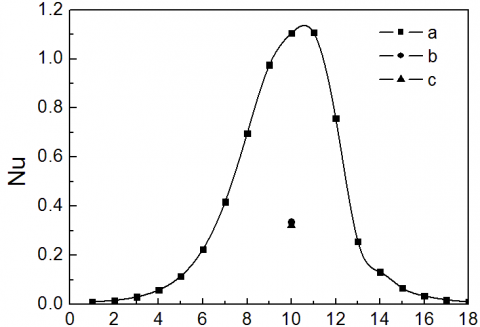(c) Lx=4

## 15d.png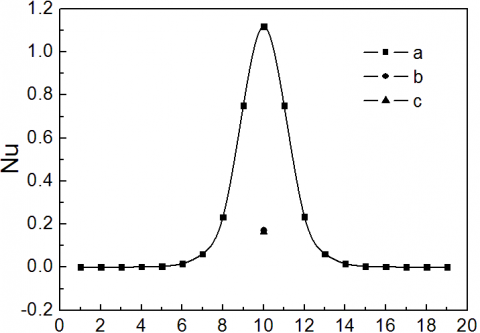(d) Lx=8

Figure 15. Average Nusselt number

4. Conclusion

Natural convection has been numerically simulated in a small cavity with one, two, or three heat sources using the SIMPLE algorithm with a QUICK scheme. The temperature field, flow field, and three-dimensional characteristics of the cavity have been investigated. The results show that the number of vortices increases and the air temperature rises with more heat sources. For lower Rayleigh number, the heat-transfer effect is better in a square cavity than in a rectangular one. The average Nusselt number at the top plate is larger when the heat source is close to the center, rather than near the boundary. Flow and heat-transfer constraints were satisfied. Heat transfer is better when the heat source is close to the center rather than near the boundary. The X=Lx/2 temperature fields are similar to two-dimensional temperature fields. The three-dimensional model can therefore be replaced by a two-dimensional model.

Acknowledgement

This work was supported by the National Natural Science Foundation of China (Grant No. 51206061) and the Natural Science Foundation of the Jilin Provincial Science & Technology Department (Grant No. 20130101073JC).

References

1. Y. B. Liu, Research on Heat Dissipation Technique for Electronic Equipment, Electronics Process Technology, vol. 28(5), pp. 286-291, 2007.

2. L. Guo, Development of Heat Dissipation in Electronics Components [J], Refrigeration, vol. 42(10), pp. 62-66, 2013.

3. J. R. Chen, M. B. Zhu, Application of Icepak in the Thermal Design of an Electronic Device [J], Electro- Mechanical Engineering, vol. 21(1), pp. 14-16, 2005.

4. H. H. Yang, Electronic Heat Removing and  New Cooling Technology [J], New Technology & New Process, vol.5, pp. 71-72, 2006.

5. X. M. Hai, Q. W. Wang, Numerical Analysis of Closed Square Cavity Size Multi-Block Square Cavity Isolated Natural Convection Heat Transfer [J], Journal of Engineering Thermophysics, vol. 20(11), pp. 742-745, 1999.

6. Y. H. Man, J. J. Mi, A Numerical Study on Three- dimensional Conjugate Heat Transfer of Natural Convection and Conduction in a Differently Heated Cubic Enclosure with a Heat-generating Cubic Conducting Body [J], Int J Heat Mass Transfer, vol. 43, pp. 4229-4248, 2000. DOI:  10.1016/S0017- 9310(00)00063-6.

7. W. Yang, J. G. Chang, Effects of Air Parameters on Numerical Simulation of Natural Convection in a Closed Square Cavity [J], Chinese Quarterly of Mechanics, vol. 34(12), pp. 630-635, 2013.

8. S. S. Song, X. Y. Guo, Bousinesq Approximation and Numerical Simulation of Natural Convection in a Closed Square Cavity [J], Chinese Quarterly of Mechanics, vol. 33(1), pp. 60-67, 2012.

9. C. H. Pei, F. Wu, Numerical Simulation of Convective Heat Transfer Under Periodic Temperature Boundary Unilateral Square Cavity Natural Porous Media [J], Journal of Xi’an Shiyou University (Natural Science Edition), vol. 29(5), pp. 73-78, 2014.

10. K. D. Manab, K. S. K. Saran, Conjugate Natural Convection Heat Transfer in an Inclined Square Cavity Containing a Conducting Block [J], Int J Heat Mass Transfer, vol. 49, pp. 4987-5000, 2006. DOI: 10.1016/j.ijheatmasstransfer.2006.05.041.

11. Sharif M. A. R., Laminar Mixed Convection in Shallow Inclined Driven Cavity with Hot Moving Lid on Top and Cooled from Bottom [J], Apply Therm Eng, vol. 27, pp. 1036-1042, 2007. Doi: 10.1016/j.applthermaleng.2006.07.035.

12. S. F. Dong , Y. T. Li, Conjugate of Natural Convection and Conduction in a Complicated Enclosure [J], Int J Heat Mass Transfer, vol. 47, pp. 2233-2239, 2004. Doi: 10.1016/j.ijheatmasstransfer.2003.11.018.

13. Q. W. Wang, et al., Experimental Study on Natural Convection in a Inclined Square Cavity [J], Journal of Engineering Thermophysics, vol. 19(20), pp. 204-208, 1998.

14. H. L. Jiang, Y.G. Zhou, Study on  Natural Convection in a Rectangular Cavity with Heat Sources, Xi’an Jiaotong University Xuebao, vol. 32(11), pp. 40-43,1998.

15. Markatos K. A. Pericleous, Laminar and Turbulent Natural Convection in an Enclosed Cavity, Int J Heat Mass Transfer, vol. 27(5), pp. 755-772, 1984. Doi: 10.1016/0017-9310(84)90145-5.